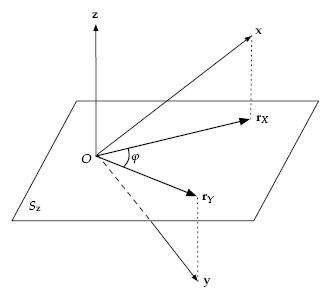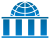# Partial correlation

Not to be confused with Coefficient of partial determination.

In probability theory and statistics, partial correlation measures the degree of association between two random variables, with the effect of a set of controlling random variables removed.

## Formal definition

Formally, the partial correlation between X and Y given a set of n controlling variables Z = {Z1, Z2, ..., Zn}, written ρXY·Z, is the correlation between the residuals RX and RY resulting from the linear regression of X with Z and of Y with Z, respectively. The first-order partial correlation (i.e. when n=1) is the difference between a correlation and the product of the removable correlations divided by the product of the coefficients of alienation of the removable correlations. The coefficient of alienation, and its relation with joint variance through correlation are available in Guilford (1973, pp. 344–345).

## Computation

### Using linear regression

A simple way to compute the sample partial correlation for some data is to solve the two associated linear regression problems, get the residuals, and calculate the correlation between the residuals. Let X and Y be, as above, random variables taking real values, and let Z be the n-dimensional vector-valued random variable. If we write xi, yi and zi to denote the ith of N i.i.d. samples of some joint probability distribution over real random variables X, Y and Z, solving the linear regression problem amounts to finding n-dimensional coefficient vectorsandsuch thatwith N being the number of samples andthe scalar product between the vectors v and w. Note that in some formulations the regression includes a constant term, so the matrixwould have an additional column of ones.

The residuals are thenand the sample partial correlation is then given by the usual formula for sample correlation , but between these new derived values.### Using recursive formula

It can be computationally expensive to solve the linear regression problems. Actually, the nth-order partial correlation (i.e., with |Z| = n) can be easily computed from three (n - 1)th-order partial correlations. The zeroth-order partial correlation ρXY·Ø is defined to be the regular correlation coefficient ρXY.

It holds, for any:Naïvely implementing this computation as a recursive algorithm yields an exponential time complexity. However, this computation has the overlapping subproblems property, such that using dynamic programming or simply caching the results of the recursive calls yields a complexity of.

Note in the case where Z is a single variable, this reduces to:### Using matrix inversion

Intime, another approach allows all partial correlations to be computed between any two variables Xi and Xj of a set V of cardinality n, given all others, i.e.,, if the correlation matrix (or alternatively covariance matrix) Ω = (ωij), where ωij = ρXiXj, is positive definite and therefore invertible. If we define P = Ω−1, we have:## Interpretation### Geometrical

Let three variables X, Y, Z (where Z is the "control" or "extra variable") be chosen from a joint probability distribution over n variables V. Further let vi, 1 ≤ iN, be N n-dimensional i.i.d. samples taken from the joint probability distribution over V. We then consider the N-dimensional vectors x (formed by the successive values of X over the samples), y (formed by the values of Y) and z (formed by the values of Z).

It can be shown that the residuals RX coming from the linear regression of X on Z, if also considered as an N-dimensional vector rX, have a zero scalar product with the vector z generated by Z. This means that the residuals vector lies on an (N–1)-dimensional hyperplane Sz that is perpendicular to z.

The same also applies to the residuals RY generating a vector rY. The desired partial correlation is then the cosine of the angle φ between the projections rX and rY of x and y, respectively, onto the hyperplane perpendicular to z.:ch. 7

### As conditional independence test

With the assumption that all involved variables are multivariate Gaussian, the partial correlation ρXY·Z is zero if and only if X is conditionally independent from Y given Z. This property does not hold in the general case.

To test if a sample partial correlationvanishes, Fisher's z-transform of the partial correlation can be used:The null hypothesis is, to be tested against the two-tail alternative. We reject H0 with significance level α if:where Φ(·) is the cumulative distribution function of a Gaussian distribution with zero mean and unit standard deviation, and N is the sample size. Note that this z-transform is approximate and that the actual distribution of the sample (partial) correlation coefficient is not straightforward. However, an exact t-test based on a combination of the partial regression coefficient, the partial correlation coefficient and the partial variances is available.

The distribution of the sample partial correlation was described by Fisher.

## Semipartial correlation (part correlation)

The semipartial (or part) correlation statistic is similar to the partial correlation statistic. Both compare variations of two variables after certain factors are controlled for, but to calculate the semipartial correlation one holds the third variable constant for either X or Y but not both, whereas for the partial correlation one holds the third variable constant for both. The semipartial correlation compares the unique variation of one variable (having removed variation associated with the Z variable(s)), with the unfiltered variation of the other, while the partial correlation compares the unique variation of one variable to the unique variation of the other.

The semipartial (or part) correlation can be viewed as more practically relevant "because it is scaled to (i.e., relative to) the total variability in the dependent (response) variable."  Conversely, it is less theoretically useful because it is less precise about the role of the unique contribution of the independent variable.

The absolute value of the semipartial correlation of X with Y is always less than or equal to that of the partial correlation of X with Y. The reason is this: Suppose the correlation of X with Z has been removed from X, giving the residual vector rx . In computing the semipartial correlation, Y still contains both unique variance and variance due to its association with Z. But rx , being uncorrelated with Z, can only explain some of the unique part of the variance of Y and not the part related to Z. In contrast, with the partial correlation, only ry (the part of the variance of Y that is unrelated to Z) is to be explained, so there is less variance of the type that rx cannot explain.

## Use in time series analysis

In time series analysis, the partial autocorrelation function (sometimes "partial correlation function") of a time series is defined, for lag h, asThis function is used to determine the appropriate lag length for an autoregression.

6. http://luna.cas.usf.edu/~mbrannic/files/regression/Partial.html. Missing or empty |title= (help)Wikiversity has learning materials about Partial correlation